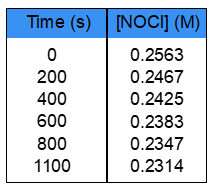Clutch Prep is now a part of Pearson
Ch.13 - Chemical KineticsWorksheetSee all chapters

# Half-Life

See all sections
Sections
Intro to Chemical Kinetics
Energy Diagrams
Catalyst
Factors Influencing Rates
Average Rate of Reaction
Stoichiometric Rate Calculations
Instantaneous Rate
Collision Theory
Arrhenius Equation
Rate Law
Reaction Mechanism
Integrated Rate Law
Half-Life

Half-Life is the time it takes for half of a reactant to decay in a certain time period.

###### Understanding Half-Life

Concept #1: Zero-Order Half-Life

Example #1: The reverse Haber reaction: 2 NH4 (g) → 3 H2 (g) + N2 (g), has a rate constant of 1.45 x 10-6 M•s-1 at 25ºC. Calculate the half-life for the reaction where [NH4]0 = 2.47 x 10-2 mol/L.

Practice: Decomposition of a certain substance Y at 45°C was found to be zero order. What is the half-life of substance Y if it took 15.5 minutes to decompose 67% of this substance? [Y]0 = 0.25 M.

Concept #2: First-Order Half-Life

Example #2: Rate constant for the following reaction was found to be 2.3 x 10-3 s-1 at 35ºC:

2 NO→ N2O4

If the initial concentration of NO2 was 1.4 x 10-1, what is the half-life on this reaction?

Practice: Radioactive plutonium-239 (t1/2 = 2.41 × 105 yr) is used in nuclear reactors and atomic bombs. If there are 5.70 × 102 g of plutonium isotope in a small atomic bomb, how long will it take for the substance to decay to 3.00 × 102 g?

Practice: Which of the following statements is False?

Concept #3: Second-Order Half-Life

Example #3: The half-life of a certain reaction with 2nd order was found to be 0.45 seconds. What was the initial concentration of a reactant if the slope of the straight line for this reaction is 3.5 x 10-3?

Practice: Use the data below to determine the half-life of decomposition of NOCl reaction which follows 2nd order kinetics.